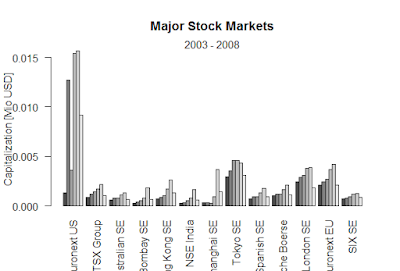Showing posts with label CMFI. Show all posts
Showing posts with label CMFI. Show all posts

## Chaikin Money Flow Index

Chicken money flow index is a volume-based indicator it is developed by mark checking this is another indicator that combines the price movement with the volume the price ending the day close to the days hi source the strength of the bulls while if it happens at the lower end of the range it reveals the strength of the bears.
Calculation
Checking money flow index is calculated using the three-step process and the formula to calculate for 10 days CMFI
1. Money flow multiplier={(Close-low)-(high-close)}/(high-low)
2. Money flow volume=Money flow multiplier*volume for the period
3.10 period CMFI=10period sum of money flow volume/10 period sum of volume.
The money flow multiplier tries to identify where the closing takes place. if it is above the halfway mark of high low range the money flow multiplied will be positive otherwise it will be negative. The money flow multiplier will be+1 if the closing occurs at high and-1 if the closing occurs at a low.all you have to do now is to calculate the weighted average of these money flow x using the volume as weight. The last two points are given in the formula explain this throw you can compute CMFI for in time period the commonly used one of the 21 one is 21 days which is typically the number trading days in a month.
Interpretation
This combination of volume price helps checking the money flow index to determine the strength of police or varies move in an indicator seconds formula such that the interpretations of this indicator becomes easier. Answer reading table of 0 is treated as police reading below zero is stated as virus.the level of the oxalates shoes the strength of police obvious pressure. Example checking money flow index reading AVN + 0.25 or below -0.25 indicates that the police baras favor is getting too much. In other words the counter is in the overbought oversold situation and main rivers the directions.

### Black-Scholes formula-R

Black-Scholes formula-R > BlackScholes <- function(TypeFlag = c("c", "p"), S, X, Time, r, b, sigma) { TypeFla...